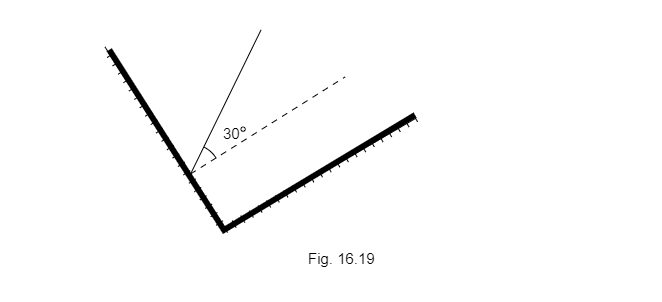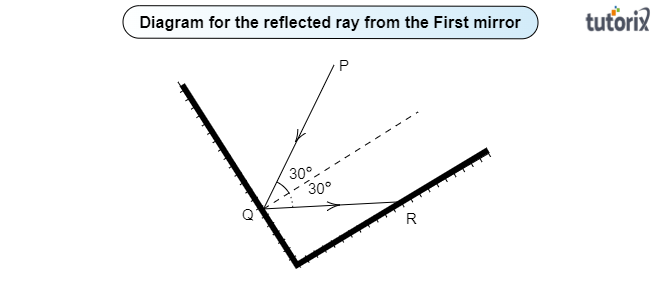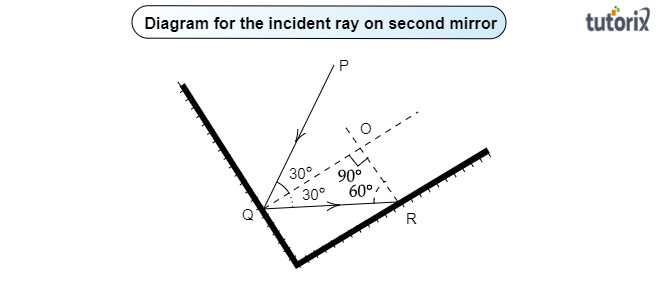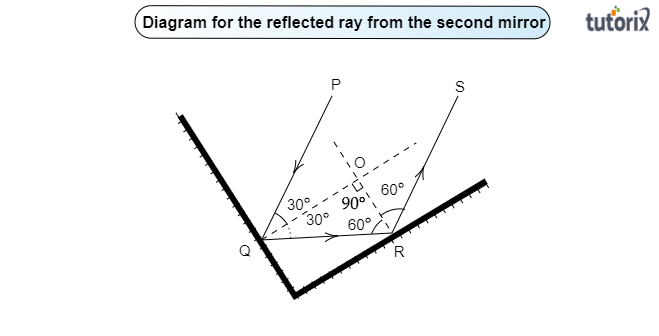# Two mirrors meet at right angles. A ray of light is incident on one at an angle of $30^{\circ}$ as shown in Fig. 16.19. Draw the reflected ray from the second mirror."

Given:

Two mirrors meet at right angles and a ray of light is incident on one at an angle of $30^{\circ}$ as shown in Fig. 16.19.

To do:

To draw the reflected ray from the second mirror.

Solution:

Before drawing the reflected ray from the second mirror, let us observe the given fig. 16.19 and draw the reflected ray from the first mirror.

Incident ray on the first mirror and reflected ray from the first mirror:

Let us assume that a ray PQ is an incident on the first mirror at an angle of $30^{\circ}$ to the normal. Here $30^{\circ}$ is the angle of incidence.

According to the law of reflection of a light ray from a plane surface-

Angle of reflection$=$Angle of incidence$=30^{\circ}$

QR is the reflected ray from the first mirror at an angle of $30^{\circ}$ as shown in the figure below:Incident ray on the second mirror and reflected ray from the second mirror:
Reflected ray QR from the first mirror is the incident ray for the second mirror. Let us find the angle of incidence of the ray QR by drawing a normal on the second mirror at R:Both normals intersect at O at the angle of $90^{\circ}$. Let's use the angle sum property of a triangle in $\triangle OQR$ to find the $\angle$ R:

$\angle$ Q$+\angle R+\angle$O$=180^{\circ}$

Or $30^{\circ}+\angle$ R$+90^{\circ}=180^{\circ}$

Or $\angle$ R$=180^{\circ}-(30^{\circ}+90^{\circ})$

Or $\angle$R$=60^{\circ}$

Therefore, the angle of incidence in the second mirror $=60^{\circ}$

As per the law of reflection, the angle of reflection from the second mirror$=60^{\circ}$

RS is the reflected ray from the second mirror as shown in the diagram below: